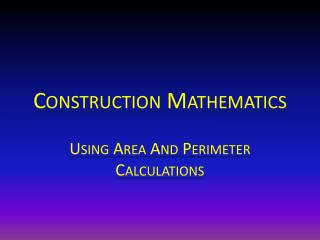Download PresentationConstruction Mathematics# Construction Mathematics - PowerPoint PPT Presentation

Download Presentation##### Construction Mathematics

Download Policy: Content on the Website is provided to you AS IS for your information and personal use and may not be sold / licensed / shared on other websites without getting consent from its author. While downloading, if for some reason you are not able to download a presentation, the publisher may have deleted the file from their server.

- - - - - - - - - - - - - - - - - - - - - - - - - - - E N D - - - - - - - - - - - - - - - - - - - - - - - - - - -
##### Presentation Transcript

1. Construction Mathematics Using Area And Perimeter Calculations

2. Brainstorm: How much base molding is required for this room? What formula would you use? Brainstorm: What other type of construction mathematic applications could this be used for?

3. Brainstorm: What areas would you break this shape up into to calculate the floor area minus the corner fireplace?

4. Let’s Discuss It Solution: Using the formula for perimeter we will add all sides together to determine the linear feet of molding needed. S1= 5’ +3’+5’=13’ S2-6= 9’+10’+8’+10’+9’= 46’ 46’+13’=59’ This application could also be used to calculate for Wall paper border or crown molding.

5. SOLUTION: The area could be broken down as follows A D C B Area A: Triangle Area B : Square Area C; Rectangle Area D: ½ Circle

6. Formulas Circle: pie r squared BUT IT IS A HALF CIRCLE SO DIVIDE BY 2

7. SQUARE & RECTANGLE SQUARE AND RECTANGLE ARE BOTH LENGTH X WIDTH

8. TRIANGLE ½ BASE X HEIGHT

9. IN CONCLUSION USING BASIC MATH CALCULATIONS ITEMS SUCH AS THE FOLLOWING CAN BE ESTIMATED FOR CONSTRUCTION WALL AREA MOLDING LENGTH FLOOR AREA CEILING AREA